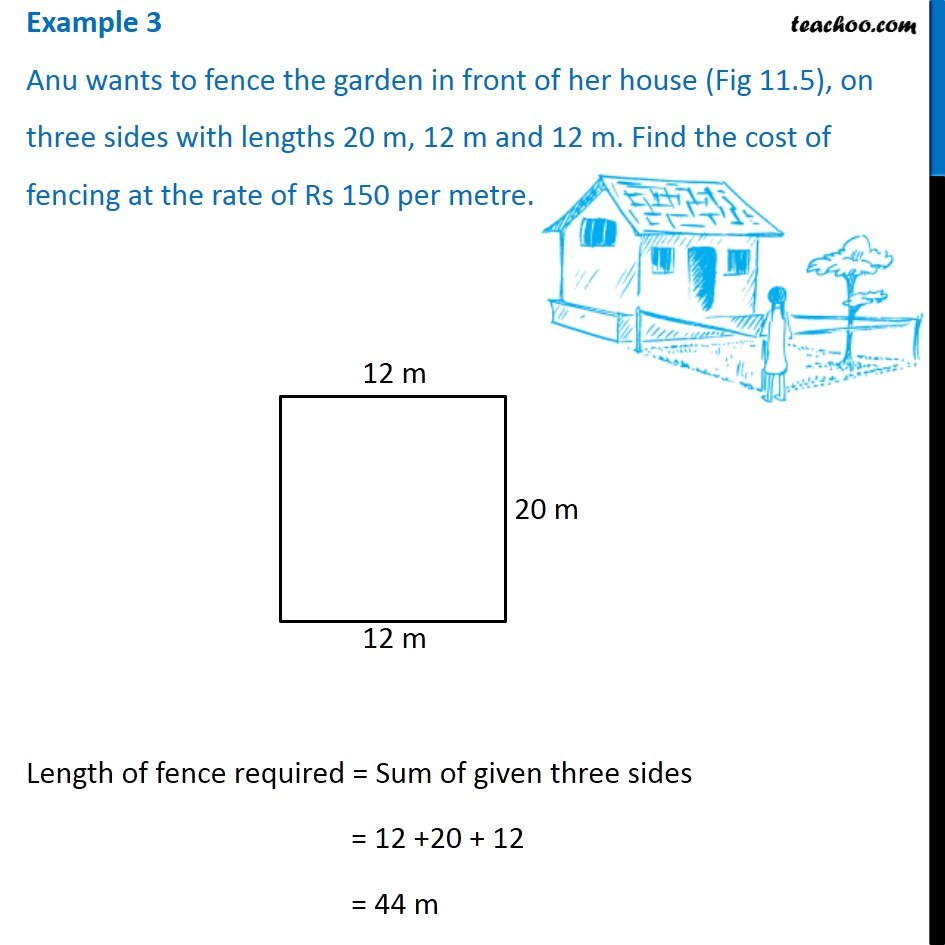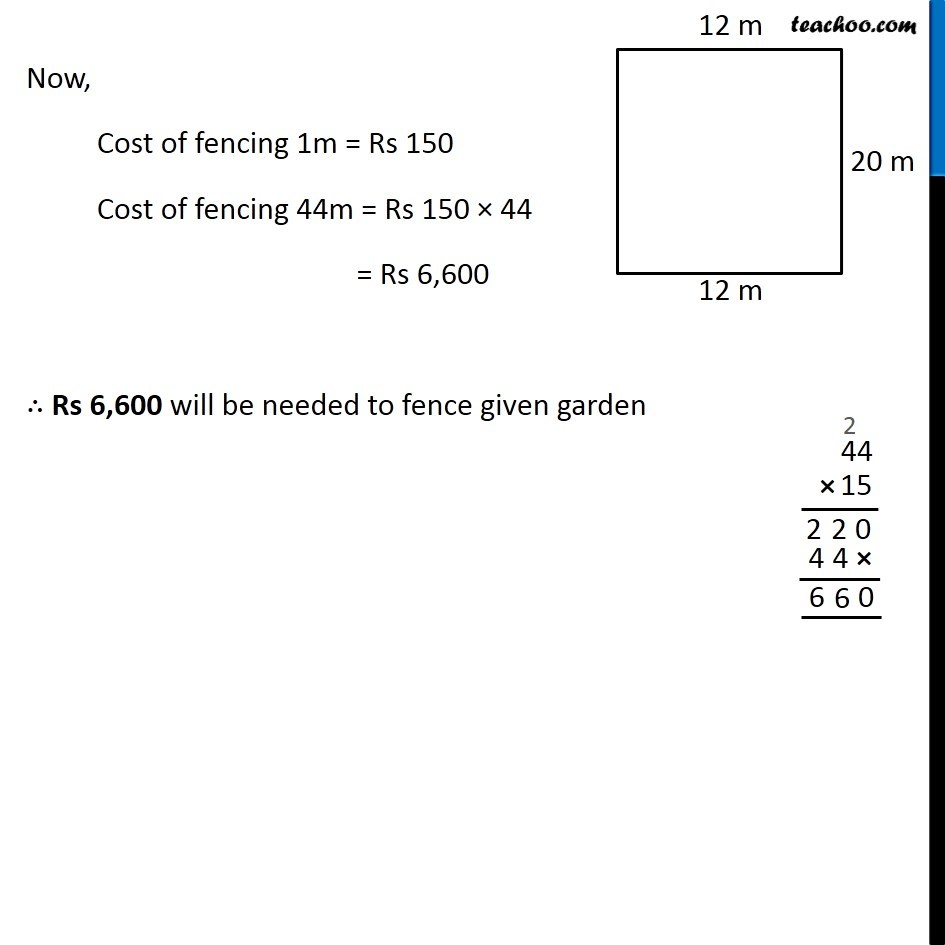1. Chapter 11 Class 7 Perimeter and Area
2. Serial order wise
3. Examples

Transcript

Example 3 Anu wants to fence the garden in front of her house (Fig 11.5), on three sides with lengths 20 m, 12 m and 12 m. Find the cost of fencing at the rate of Rs 150 per metre. Length of fence required = Sum of given three sides = 12 +20 + 12 = 44 m Now, Cost of fencing 1m = Rs 150 Cost of fencing 44m = Rs 150 × 44 = Rs 6,600 ∴ Rs 6,600 will be needed to fence given garden